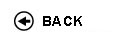Politecnico di Torino
Academic Year 2009/10
19ACIFD, 19ACIEU, 19ACIEX, 19ACIFN
Mathematical analysis II
1st degree and Bachelor-level of the Bologna process in Electrical Engineering - Torino
1st degree and Bachelor-level of the Bologna process in Chemical Engineering - Torino
1st degree and Bachelor-level of the Bologna process in Material Engineering - Torino
Espandi...
 Teacher Status SSD Les Ex Lab Tut Years teaching Nicola Fabio O2 MAT/05 48 36 0 0 10
 SSD CFU Activities Area context MAT/05 7.5 A - Di base Matematica, informatica e statistica
 Objectives of the course Main goal of this course is to complete the introduction of the basic topics of mathematical analysis of functions of several variables. In particular, the theory of multiple integration and of surface integration is developed. Furthermore, numerical series and series of functions (power series and Fourier series in particular) and systems of ordinary differential equations are studied. Expected skills Familiarity with the subjects of the course and computational ability. Ability to read a technical text. Prerequisites The topics of the courses Analisi Matematica 1 and Geometria. In particular: limits of functions and differential calculus for functions of one variable; integrals of functions of one variable and differential equations; linear algebra, eigenvalues and eigenvectors; geometry of curves and surfaces. Continuity of functions of several variables, computation of partial derivatives, differential and jacobian matrix. Minima and maxima of functions of several variables, taken at interior points of the domain. Syllabus Differential calculus of functions of several variables: implicit functions; Lagrange multipliers; inverse function theorem and transformations of coordinate systems. Integral calculus: multiple integration. Length of a curve and area of a surface. Curve and surface integration. Green, Gauss and Stokes Theorems. Conservative fields and differential forms. Numerical series and series of functions: definition and criteria for convergence. Power series and Taylor series. Series solutions of ordinary differential equations of the first order. Fourier series in real and complex form: computation of the coefficients and convergence properties. Systems of differential equations: solutions of linear systems. Qualitative behaviour of the solutions. Laboratories and/or exercises Exercises will be made in class. Bibliography The following texts are used in the courses of Analisi Matematica 2. Ask your teacher or look at the WEB page of your course for specific information. C. Canuto, A. Tabacco, Analisi Matematica II, Sprinter J. P. Cecconi, G. Stampacchia, Analisi Matematica 2, Liguori N. Fusco, F. Marcellini, C. Sbordone, Elementi di Analisi Matematica due, Liguori Ed. M. Bramanti, C.D. Pagani, S. Salsa: Matematica, Calcolo infinitesimale e algebra lineare, seconda edizione, Zanichelli L. Pandolfi, Lezioni di Analisi Matematica 2 (can be freely downloaded from 'Portale della didattica') A. Bacciotti, P. Boieri, D. Farina: Esercizi di calcolo differenziale e integrale in piů variabili, Societŕ editrice Esculapio. D.Bazzanella, P.Boieri, L.Caire, A.Tabacco, Serie di funzioni e trasformate, Teoria ed Esercizi, Clut. V. Demidovich, Esercizi e problemi di Analisi Matematica, Editori Riuniti F. Marcellini, C. Sbordone, Esercitazioni di matematica, secondo volume prima e seconda parte, Liguori Ed. S. Salsa - A. Squellati: Esercizi di Analisi Matematica 2, Parte prima, seconda, terza, Masson. Check availability at the library Revisions / Exam The exam consists of a written and of an oral part. The written part ascertains the familiarity of the student with the arguments of the course while the oral part is mostly concerned with the theory. In order to be admitted to the oral part of the exam, students should score 15/30 or higher in the written part. Programma definitivo per l'A.A.2010/11© Politecnico di Torino
Corso Duca degli Abruzzi, 24 - 10129 Torino, ITALY